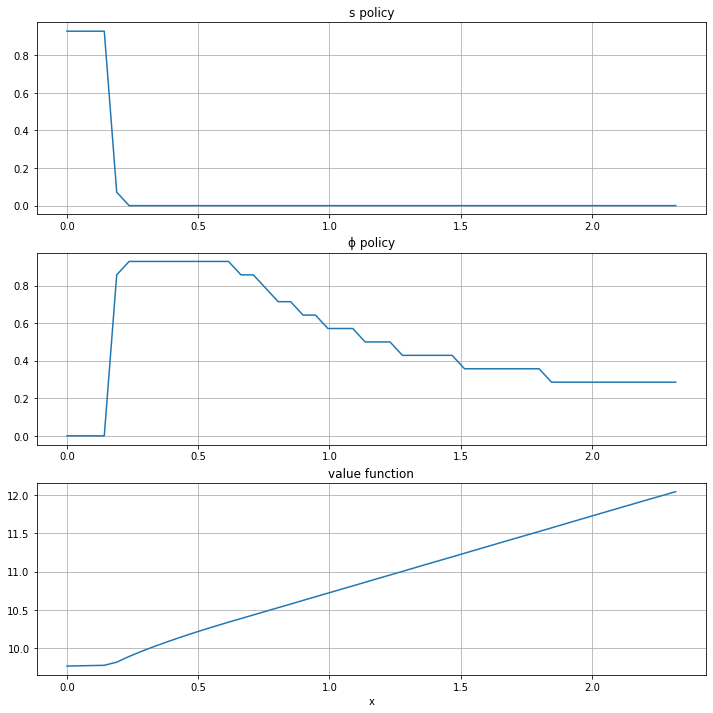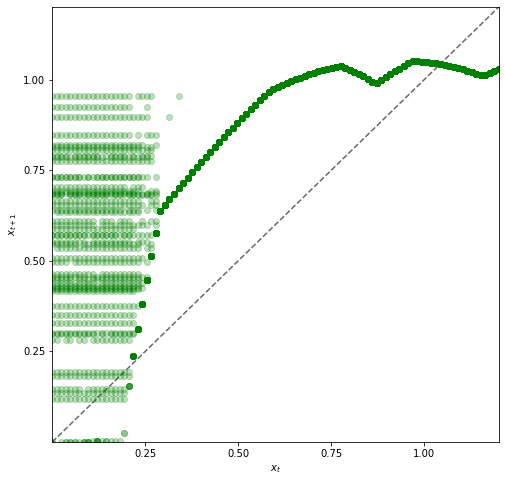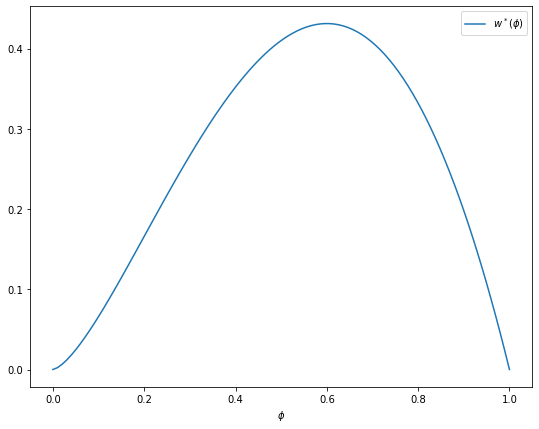# Job Search VI: On-the-Job Search¶

## Contents¶

In addition to what’s in Anaconda, this lecture will need the following libraries:

In :
!pip install quantecon
!pip install interpolation


## Overview¶

In this section, we solve a simple on-the-job search model

Let’s start with some imports:

In :
import numpy as np
import scipy.stats as stats
from interpolation import interp
from numba import njit, prange
import matplotlib.pyplot as plt
%matplotlib inline
from math import gamma


### Model Features¶

• job-specific human capital accumulation combined with on-the-job search
• infinite-horizon dynamic programming with one state variable and two controls

## Model¶

Let $x_t$ denote the time-$t$ job-specific human capital of a worker employed at a given firm and let $w_t$ denote current wages.

Let $w_t = x_t(1 - s_t - \phi_t)$, where

• $\phi_t$ is investment in job-specific human capital for the current role and
• $s_t$ is search effort, devoted to obtaining new offers from other firms.

For as long as the worker remains in the current job, evolution of $\{x_t\}$ is given by $x_{t+1} = g(x_t, \phi_t)$.

When search effort at $t$ is $s_t$, the worker receives a new job offer with probability $\pi(s_t) \in [0, 1]$.

The value of the offer, measured in job-specific human capital, is $u_{t+1}$, where $\{u_t\}$ is IID with common distribution $f$.

The worker can reject the current offer and continue with existing job.

Hence $x_{t+1} = u_{t+1}$ if he/she accepts and $x_{t+1} = g(x_t, \phi_t)$ otherwise.

Let $b_{t+1} \in \{0,1\}$ be a binary random variable, where $b_{t+1} = 1$ indicates that the worker receives an offer at the end of time $t$.

We can write

$$x_{t+1} = (1 - b_{t+1}) g(x_t, \phi_t) + b_{t+1} \max \{ g(x_t, \phi_t), u_{t+1}\} \tag{1}$$

Agent’s objective: maximize expected discounted sum of wages via controls $\{s_t\}$ and $\{\phi_t\}$.

Taking the expectation of $v(x_{t+1})$ and using (1), the Bellman equation for this problem can be written as

$$v(x) = \max_{s + \phi \leq 1} \left\{ x (1 - s - \phi) + \beta (1 - \pi(s)) v[g(x, \phi)] + \beta \pi(s) \int v[g(x, \phi) \vee u] f(du) \right\} \tag{2}$$

Here nonnegativity of $s$ and $\phi$ is understood, while $a \vee b := \max\{a, b\}$.

### Parameterization¶

In the implementation below, we will focus on the parameterization

$$g(x, \phi) = A (x \phi)^{\alpha}, \quad \pi(s) = \sqrt s \quad \text{and} \quad f = \text{Beta}(2, 2)$$

with default parameter values

• $A = 1.4$
• $\alpha = 0.6$
• $\beta = 0.96$

The $\text{Beta}(2,2)$ distribution is supported on $(0,1)$ - it has a unimodal, symmetric density peaked at 0.5.

### Back-of-the-Envelope Calculations¶

Before we solve the model, let’s make some quick calculations that provide intuition on what the solution should look like.

To begin, observe that the worker has two instruments to build capital and hence wages:

1. invest in capital specific to the current job via $\phi$
2. search for a new job with better job-specific capital match via $s$

Since wages are $x (1 - s - \phi)$, marginal cost of investment via either $\phi$ or $s$ is identical.

Our risk-neutral worker should focus on whatever instrument has the highest expected return.

The relative expected return will depend on $x$.

For example, suppose first that $x = 0.05$

• If $s=1$ and $\phi = 0$, then since $g(x,\phi) = 0$, taking expectations of (1) gives expected next period capital equal to $\pi(s) \mathbb{E} u = \mathbb{E} u = 0.5$.
• If $s=0$ and $\phi=1$, then next period capital is $g(x, \phi) = g(0.05, 1) \approx 0.23$.

Both rates of return are good, but the return from search is better.

Next, suppose that $x = 0.4$

• If $s=1$ and $\phi = 0$, then expected next period capital is again $0.5$
• If $s=0$ and $\phi = 1$, then $g(x, \phi) = g(0.4, 1) \approx 0.8$

Return from investment via $\phi$ dominates expected return from search.

Combining these observations gives us two informal predictions:

1. At any given state $x$, the two controls $\phi$ and $s$ will function primarily as substitutes — worker will focus on whichever instrument has the higher expected return.
2. For sufficiently small $x$, search will be preferable to investment in job-specific human capital. For larger $x$, the reverse will be true.

Now let’s turn to implementation, and see if we can match our predictions.

## Implementation¶

We will set up a class JVWorker that holds the parameters of the model described above

In :
class JVWorker:
r"""
A Jovanovic-type model of employment with on-the-job search.

"""

def __init__(self,
A=1.4,
α=0.6,
β=0.96,         # Discount factor
π=np.sqrt,      # Search effort function
a=2,            # Parameter of f
b=2,            # Parameter of f
grid_size=50,
mc_size=100,
ɛ=1e-4):

self.A, self.α, self.β, self.π = A, α, β, π
self.mc_size, self.ɛ = mc_size, ɛ

self.g = njit(lambda x, ϕ: A * (x * ϕ)**α)    # Transition function
self.f_rvs = np.random.beta(a, b, mc_size)

# Max of grid is the max of a large quantile value for f and the
# fixed point y = g(y, 1)
ɛ = 1e-4
grid_max = max(A**(1 / (1 - α)), stats.beta(a, b).ppf(1 - ɛ))

# Human capital
self.x_grid = np.linspace(ɛ, grid_max, grid_size)


The function operator_factory takes an instance of this class and returns a jitted version of the Bellman operator T, i.e.

$$Tv(x) = \max_{s + \phi \leq 1} w(s, \phi)$$

where

$$w(s, \phi) := x (1 - s - \phi) + \beta (1 - \pi(s)) v[g(x, \phi)] + \beta \pi(s) \int v[g(x, \phi) \vee u] f(du) \tag{3}$$

When we represent $v$, it will be with a NumPy array v giving values on grid x_grid.

But to evaluate the right-hand side of (3), we need a function, so we replace the arrays v and x_grid with a function v_func that gives linear interpolation of v on x_grid.

Inside the for loop, for each x in the grid over the state space, we set up the function $w(z) = w(s, \phi)$ defined in (3).

The function is maximized over all feasible $(s, \phi)$ pairs.

Another function, get_greedy returns the optimal choice of $s$ and $\phi$ at each $x$, given a value function.

In :
def operator_factory(jv, parallel_flag=True):

"""
Returns a jitted version of the Bellman operator T

jv is an instance of JVWorker

"""

π, β = jv.π, jv.β
x_grid, ɛ, mc_size = jv.x_grid, jv.ɛ, jv.mc_size
f_rvs, g = jv.f_rvs, jv.g

@njit
def state_action_values(z, x, v):
s, ϕ = z
v_func = lambda x: interp(x_grid, v, x)

integral = 0
for m in range(mc_size):
u = f_rvs[m]
integral += v_func(max(g(x, ϕ), u))
integral = integral / mc_size

q = π(s) * integral + (1 - π(s)) * v_func(g(x, ϕ))
return x * (1 - ϕ - s) + β * q

@njit(parallel=parallel_flag)
def T(v):
"""
The Bellman operator
"""

v_new = np.empty_like(v)
for i in prange(len(x_grid)):
x = x_grid[i]

# Search on a grid
search_grid = np.linspace(ɛ, 1, 15)
max_val = -1
for s in search_grid:
for ϕ in search_grid:
current_val = state_action_values((s, ϕ), x, v) if s + ϕ <= 1 else -1
if current_val > max_val:
max_val = current_val
v_new[i] = max_val

return v_new

@njit
def get_greedy(v):
"""
Computes the v-greedy policy of a given function v
"""
s_policy, ϕ_policy = np.empty_like(v), np.empty_like(v)

for i in range(len(x_grid)):
x = x_grid[i]
# Search on a grid
search_grid = np.linspace(ɛ, 1, 15)
max_val = -1
for s in search_grid:
for ϕ in search_grid:
current_val = state_action_values((s, ϕ), x, v) if s + ϕ <= 1 else -1
if current_val > max_val:
max_val = current_val
max_s, max_ϕ = s, ϕ
s_policy[i], ϕ_policy[i] = max_s, max_ϕ
return s_policy, ϕ_policy

return T, get_greedy


To solve the model, we will write a function that uses the Bellman operator and iterates to find a fixed point.

In :
def solve_model(jv,
use_parallel=True,
tol=1e-4,
max_iter=1000,
verbose=True,
print_skip=25):

"""
Solves the model by value function iteration

* jv is an instance of JVWorker

"""

T, _ = operator_factory(jv, parallel_flag=use_parallel)

# Set up loop
v = jv.x_grid * 0.5  # Initial condition
i = 0
error = tol + 1

while i < max_iter and error > tol:
v_new = T(v)
error = np.max(np.abs(v - v_new))
i += 1
if verbose and i % print_skip == 0:
print(f"Error at iteration {i} is {error}.")
v = v_new

if i == max_iter:
print("Failed to converge!")

if verbose and i < max_iter:
print(f"\nConverged in {i} iterations.")

return v_new


## Solving for Policies¶

Let’s generate the optimal policies and see what they look like.

In :
jv = JVWorker()
T, get_greedy = operator_factory(jv)
v_star = solve_model(jv)
s_star, ϕ_star = get_greedy(v_star)

/home/ubuntu/anaconda3/lib/python3.7/site-packages/numba/np/ufunc/parallel.py:355: NumbaWarning: The TBB threading layer requires TBB version 2019.5 or later i.e., TBB_INTERFACE_VERSION >= 11005. Found TBB_INTERFACE_VERSION = 11004. The TBB threading layer is disabled.
warnings.warn(problem)

Error at iteration 25 is 0.15111147557693805.
Error at iteration 50 is 0.054460079651871496.
Error at iteration 75 is 0.019627233906362562.
Error at iteration 100 is 0.00707359066086255.
Error at iteration 125 is 0.0025492988505728675.
Error at iteration 150 is 0.0009187589360397652.
Error at iteration 175 is 0.00033111770413540853.
Error at iteration 200 is 0.0001193337334655098.

Converged in 205 iterations.


Here are the plots:

In :
plots = [s_star, ϕ_star, v_star]
titles = ["s policy", "ϕ policy",  "value function"]

fig, axes = plt.subplots(3, 1, figsize=(12, 12))

for ax, plot, title in zip(axes, plots, titles):
ax.plot(jv.x_grid, plot)
ax.set(title=title)
ax.grid()

axes[-1].set_xlabel("x")
plt.show()The horizontal axis is the state $x$, while the vertical axis gives $s(x)$ and $\phi(x)$.

Overall, the policies match well with our predictions from above

• Worker switches from one investment strategy to the other depending on relative return.
• For low values of $x$, the best option is to search for a new job.
• Once $x$ is larger, worker does better by investing in human capital specific to the current position.

## Exercises¶

### Exercise 1¶

Let’s look at the dynamics for the state process $\{x_t\}$ associated with these policies.

The dynamics are given by (1) when $\phi_t$ and $s_t$ are chosen according to the optimal policies, and $\mathbb{P}\{b_{t+1} = 1\} = \pi(s_t)$.

Since the dynamics are random, analysis is a bit subtle.

One way to do it is to plot, for each $x$ in a relatively fine grid called plot_grid, a large number $K$ of realizations of $x_{t+1}$ given $x_t = x$.

Plot this with one dot for each realization, in the form of a 45 degree diagram, setting

jv = JVWorker(grid_size=25, mc_size=50)
plot_grid_max, plot_grid_size = 1.2, 100
plot_grid = np.linspace(0, plot_grid_max, plot_grid_size)
fig, ax = plt.subplots()
ax.set_xlim(0, plot_grid_max)
ax.set_ylim(0, plot_grid_max)


By examining the plot, argue that under the optimal policies, the state $x_t$ will converge to a constant value $\bar x$ close to unity.

Argue that at the steady state, $s_t \approx 0$ and $\phi_t \approx 0.6$.

### Exercise 2¶

In the preceding exercise, we found that $s_t$ converges to zero and $\phi_t$ converges to about 0.6.

Since these results were calculated at a value of $\beta$ close to one, let’s compare them to the best choice for an infinitely patient worker.

Intuitively, an infinitely patient worker would like to maximize steady state wages, which are a function of steady state capital.

You can take it as given—it’s certainly true—that the infinitely patient worker does not search in the long run (i.e., $s_t = 0$ for large $t$).

Thus, given $\phi$, steady state capital is the positive fixed point $x^*(\phi)$ of the map $x \mapsto g(x, \phi)$.

Steady state wages can be written as $w^*(\phi) = x^*(\phi) (1 - \phi)$.

Graph $w^*(\phi)$ with respect to $\phi$, and examine the best choice of $\phi$.

Can you give a rough interpretation for the value that you see?

## Solutions¶

### Exercise 1¶

Here’s code to produce the 45 degree diagram

In :
jv = JVWorker(grid_size=25, mc_size=50)
π, g, f_rvs, x_grid = jv.π, jv.g, jv.f_rvs, jv.x_grid
T, get_greedy = operator_factory(jv)
v_star = solve_model(jv, verbose=False)
s_policy, ϕ_policy = get_greedy(v_star)

# Turn the policy function arrays into actual functions
s = lambda y: interp(x_grid, s_policy, y)
ϕ = lambda y: interp(x_grid, ϕ_policy, y)

def h(x, b, u):
return (1 - b) * g(x, ϕ(x)) + b * max(g(x, ϕ(x)), u)

plot_grid_max, plot_grid_size = 1.2, 100
plot_grid = np.linspace(0, plot_grid_max, plot_grid_size)
fig, ax = plt.subplots(figsize=(8, 8))
ticks = (0.25, 0.5, 0.75, 1.0)
ax.set(xticks=ticks, yticks=ticks,
xlim=(0, plot_grid_max),
ylim=(0, plot_grid_max),
xlabel='$x_t$', ylabel='$x_{t+1}$')

ax.plot(plot_grid, plot_grid, 'k--', alpha=0.6)  # 45 degree line
for x in plot_grid:
for i in range(jv.mc_size):
b = 1 if np.random.uniform(0, 1) < π(s(x)) else 0
u = f_rvs[i]
y = h(x, b, u)
ax.plot(x, y, 'go', alpha=0.25)

plt.show()Looking at the dynamics, we can see that

• If $x_t$ is below about 0.2 the dynamics are random, but $x_{t+1} > x_t$ is very likely.
• As $x_t$ increases the dynamics become deterministic, and $x_t$ converges to a steady state value close to 1.

Referring back to the figure here we see that $x_t \approx 1$ means that $s_t = s(x_t) \approx 0$ and $\phi_t = \phi(x_t) \approx 0.6$.

### Exercise 2¶

The figure can be produced as follows

In :
jv = JVWorker()

def xbar(ϕ):
A, α = jv.A, jv.α
return (A * ϕ**α)**(1 / (1 - α))

ϕ_grid = np.linspace(0, 1, 100)
fig, ax = plt.subplots(figsize=(9, 7))
ax.set(xlabel='$\phi$')
ax.plot(ϕ_grid, [xbar(ϕ) * (1 - ϕ) for ϕ in ϕ_grid], label='$w^*(\phi)$')
ax.legend()

plt.show()Observe that the maximizer is around 0.6.

This is similar to the long-run value for $\phi$ obtained in exercise 1.

Hence the behavior of the infinitely patent worker is similar to that of the worker with $\beta = 0.96$.

This seems reasonable and helps us confirm that our dynamic programming solutions are probably correct.

• Share page